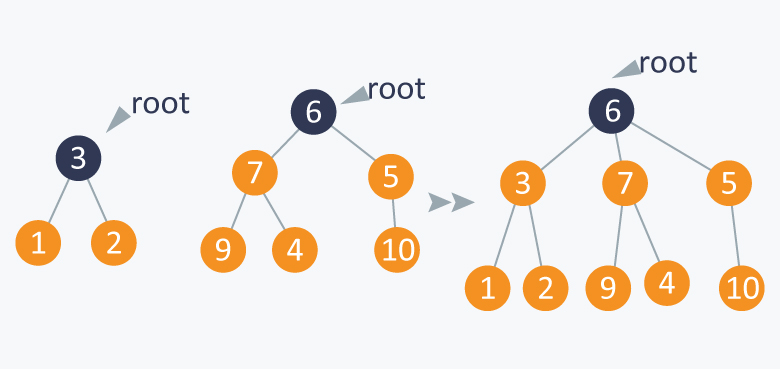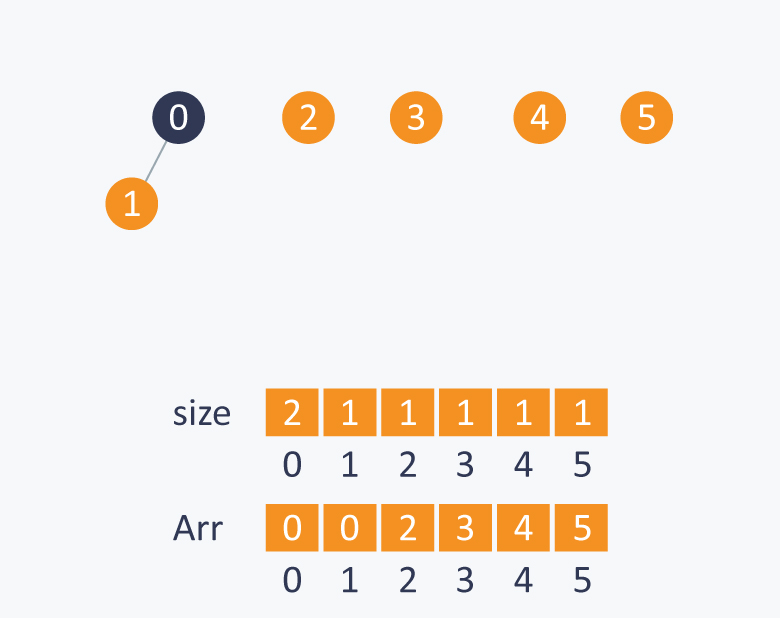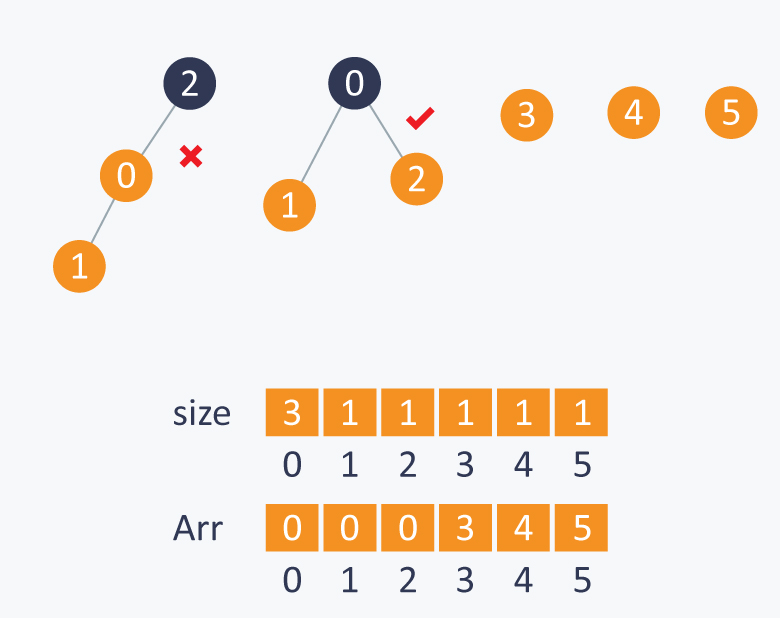# Disjoint Data Structures

To avoid disadvantages of traditional approach, track the size of each subset and while connecting two elements connect the root of each subset that has a smaller number of elements to the root of each subset that has a larger number of elements.

Example

If you want to connect 1 and 5, then connect the root of subset A (the subset that contains 1) to the root of subset B ( the subset that contains 5) because subset A contains less number of elements than subset B.It will balance the tree formed by performing the operations discussed above. This is known as weighted-union operation .

Implementation

Initially the size of each subset will be one because each subset will have only one element. You can initialize it in the initialize function discussed above. The size[ ] array function will keep a track of the size of each subset.

``````  //modified initialize function:
void initialize( int Arr[ ], int N)
{
for(int i = 0;i<N;i++)
{
Arr[ i ] = i ;
size[ i ] = 1;
}
}``````

The root() and find() functions will be same as discussed above .

The union function will be modified because the two subsets will be connected based on the number of elements in each subset.

//modified union function

``````void weighted-union(int Arr[ ],int size[ ],int A,int B)
{
int root_A = root(A);
int root_B = root(B);
if(size[root_A] < size[root_B ])
{
Arr[ root_A ] = Arr[root_B];
size[root_B] += size[root_A];
}
else
{
Arr[ root_B ] = Arr[root_A];
size[root_A] += size[root_B];
}

}``````

Example

You have a set S = {0, 1, 2, 3, 4, 5} Initially all the subsets have a single element and each element is a root of itself. Initially size[ ] array will be as follows:Perform union(0, 1). Here, you can connect any root of any element with the root of another element because both the element%u2019s subsets are of the same size. After the roots are connected, the respective sizes will be updated. If you connect 1 to 0 and make 0 the root, then the size of 0 will change from 1 to 2.While performing union(1, 2), connect root(2) with root(1) because the subset of 2 has fewer elements than the subset of 1.Similarly, in union(3, 2), connect root(3) to root(2) because the subset of 3 has fewer elements than the subset of 2.Maintaining a balance tree, will reduce complexity of the union-find function from N to log2N.

Idea for improving this approach further

Union with path compression

While computing the root of A, set each i to point to its grandparent (thereby halving the length of the path), where i is the node that comes in the path while computing the root of A.

``````//modified root function

int root (int Arr[ ] ,int i)
{
while(Arr[ i ] != i)
{
Arr[ i ] = Arr[ Arr[ i ] ] ;
i = Arr[ i ];
}
return i;
}``````

When you use the weighted-union operation with path compression it takes log * N for each union find operation, where N is the number of elements in the set.

log *N is the iterative function that computes the number of times you have to take the log of N before the value of N reaches 1.

Implementation of above approach:

#include<iostream>
using namespace std;
void initialize( int Arr[ ],int *size, int N)
{
for(int i = 0;i<N;i++)
{
Arr[ i ] = i ;
size[ i ] = 1;
}
}
//returns true if A and B are connected, else returns false
//finding root of an element
int root (int Arr[ ] ,int i)
{
while(Arr[ i ] != i)
{
Arr[ i ] = Arr[ Arr[ i ] ] ;
i = Arr[ i ];
}
return i;
}

/*modified union function where we connect the elements by changing the root of one of the elements*/

void weighted_union(int Arr[ ],int size[ ],int A,int B)
{
int root_A = root(Arr,A);
int root_B = root(Arr,B);
if(size[root_A] < size[root_B ])
{
Arr[ root_A ] = Arr[root_B];
size[root_B] += size[root_A];
}
else
{
Arr[ root_B ] = Arr[root_A];
size[root_A] += size[root_B];
}

}
bool find(int * a,int A,int B)
{
if( root(a,A)==root(a,B) ) //if A and B have the same root, it means that they are connected.
return true;
else
return false;
}
int main(){
int a,n=10;
int size;
initialize(a,size,n);
cout<<"Printing array entries:\n";
for(int i=0;i<n;i++){
cout<<a[i]<<" ";
}
cout<<endl;
cout<<"Checking whether 2 and 3 belongs to same array:\n";
if(!find(a,2,3)){
cout<<"No";
}
else{
cout<<"Yes";
}
cout<<endl;
cout<<"Making 2 and 3 to be in same group\n";
weighted_union(a,size,2,3);
cout<<"Checking whether 2 and 3 belongs to same array:\n";
if(!find(a,2,3)){
cout<<"No";
}
else{
cout<<"Yes";
}
cout<<endl;

}

Output:

```Printing array entries:
0 1 2 3 4 5 6 7 8 9
Checking whether 2 and 3 belongs to same array:
No
Making 2 and 3 to be in same group
Checking whether 2 and 3 belongs to same array:
Yes```#### More Articles of M Mounika:

Name Views Likes
C++ Segmented Sieve (Print Primes In a Range) 162 0
C++ Sieve Of Erastosthenes 135 0
C++ Gold Mine Problem 295 0
C++ Merge K Sorted Arrays 117 0
C++ K Centers Problem 240 0
C++ Find Nth Catalan Number 311 0
C++ Inplace Rotate square matrix by 90 degrees 285 0
C++ Find Non Repeating Elements in Array 86 0
C++ Merge Two Binary Trees 120 0
C++ Sum of Numbers From Root To Leaf Paths 89 0
C++ Meta Strings 91 0
C++ Flood Fill Algorithm 402 0
C++ smallest substring with maximum distinct characters 199 0
C++ Smallest window with all characters in string 93 0
C++ Minimum Removal of Characters from string to make its permutation as palindrome 86 0
C++ Minimum characters added at front of string in palindrome conversion 69 0
C++ Number of Bracket Reversals needed to make expression Balanced 72 0
C++ String to Palindrome with Append Function 83 0
C++ WildCard pattern matching 75 0
C++ Anagram substring Search 72 0
C++ Manachars Algorithm 74 0
C++ Search String in Grid 83 0
C++ String Matching(Z Algorithm) 67 0
C++ String Matching(Naive Algorithm) 113 0
C++ String Matching(KMP Algorithm) 140 0
C++ Remove Duplicates From String 110 0
C++ Basics of String Manipulation 85 1
C++ Disjoint Data Structure Cycle Detection 86 0
C++ Problem On Disjoint Data Structures 94 0
C++ Disjoint Data Structures Part3 79 0
Disjoint Data Structures Part2 90 0
Disjoint Data Structures 93 1
C++ Segment Trees 321 2
C++ Trie Cost of Data 290 1
C++ Trie Datastructure 278 1
C++ Greedy Approach Minimum number of coins 525 0
C++ Greedy Approach Maximum height Pyramid 328 1
C++ Greedy Approach String lexicographically largest subsequence 246 0
C++ Greedy Approach Lexicographically largest subsequence 364 0
C++ Greedy Approach Prims MST 398 1
C++ Greedy Approach Krushkals MST 458 1
C++ Greedy Approach N-array maximum sum 333 1
C++ Greedy Approach Policemen Catch Thieves 563 1
C++ Greedy Approach Maximum product Subset 546 1
C++ Greedy Approach Minimum Product Subset 348 1
C++ Greedy Approach Fractional Knapsack 737 1
C++ Greedy Approach-Activity Selection Problem 745 1
C++ Greedy Approach-Egyptian Fractions 639 0
C++ Greedy Approach-Huffman Codes 1031 1
C++ Introduction to Greedy Approach 955 2